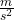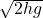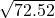Question

The best leaper in the animal kingdom is the puma, which can jump to a height of 3.7m when leaving the ground at an angle of 45 degrees. with what speed must the animal leave the ground to reach that height?

1.thugiang

v = 12 m/s

Long, boring, and convoluted explanation:

First, let’s lay out our information.

max height = 3.7 m

0 = 45°

– gravitational acceleration constant = 9.8Since the puma leaves the ground at a
45
°
angle, its motion will follow a curved path as seen in many projectile motion problems, where the object is being influenced solely by the force of gravity. And because the puma leaves the ground at an angle, its initial velocity is broken down into its horizontal and vertical components. We were also told, though indirectly, that the max height is  3.7m  because the puma can reach up to that height. Gravity is always given to be 9.8Because we are dealing with maximum height and gravity, we have to use the vertical component of the velocity,  vsin
(
θ
)

, and not the horizontal component, vcos
(
θ
)

.

Given its max height, the acceleration due to gravity, and the angle, we can now solve for the speed at which the puma leaves the ground using the following equation: vsin
(
θ
)  =Where
vsin
(
θ
)
is the vertical component of the initial velocity and h  and g are max height and gravitational acceleration constant respectively.

Plugin, rearrange and solve

v
sin
(
θ
)  =v
sin
(
45

)  =   √
2  ×  3.7  ×  9.8

v
(
0.71
)  =v
(
0.71
)  =  8.52

v  =  8.52
/0.71

v =  12
m
s